# JavaFX Transformations Tutorial with Examples

## 1- What is Transformation?

Transformation Is a geometrical concept. It means changing the shape of an object to a different shape. There are some common transformations such as:
• Translation
• Scale
• Rotation
• Shearing
• ....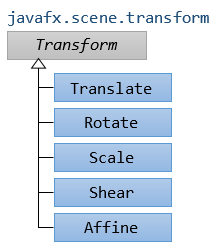Translation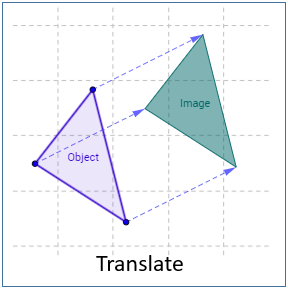Reflection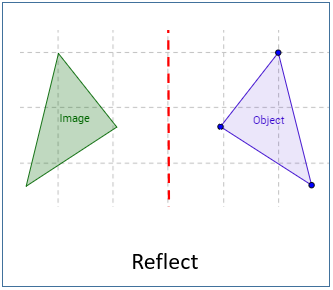Rotation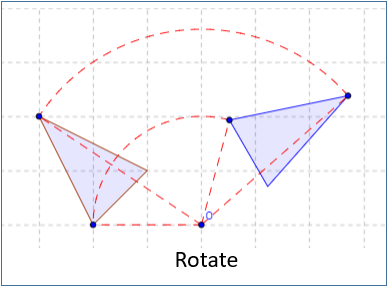Shearing/Skewing
Shear by x axis: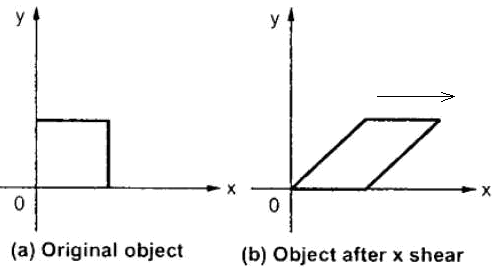Shear by y axis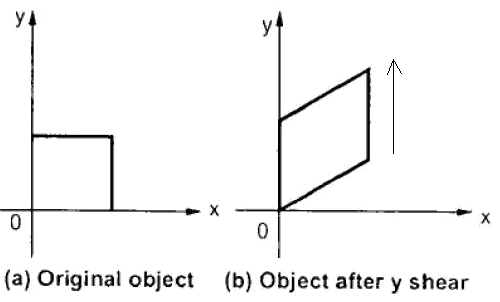## 2- Translation Transformation

Translation transformation will move an object to another location in a particular direction. The simplest example is moving one point to another location.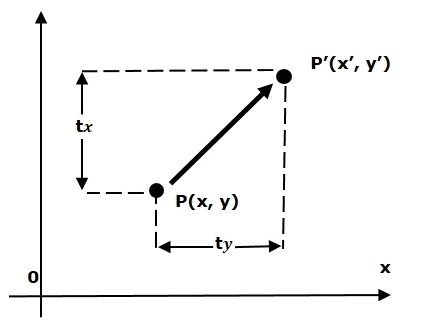All points on the geometry object move in one direction and move the same distance: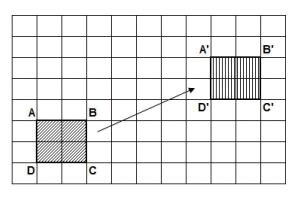Example:
Example, there are two rectangles in the same position and size, we will use the Translation Transformation to move the second rectangle. And see the result: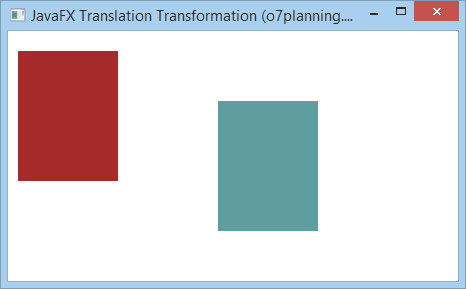TranslationExample.java
``````
package org.o7planning.javafx.transformation;

import javafx.application.Application;
import javafx.scene.Group;
import javafx.scene.Scene;
import javafx.scene.paint.Color;
import javafx.scene.shape.Rectangle;
import javafx.scene.transform.Translate;
import javafx.stage.Stage;

public class TranslationExample extends Application {

@Override
public void start(Stage stage) {

final int x = 10;
final int y = 20;
final int width = 100;
final int height = 130;

// Rectangle1
Rectangle rectangle1 = new Rectangle(x, y, width, height);

// Rectangle2 (Same position and size to rectangle1)
Rectangle rectangle2 = new Rectangle(x, y, width, height);

rectangle1.setFill(Color.BROWN);
rectangle1.setStrokeWidth(20);

rectangle2.setStrokeWidth(20);

// Creating the translation transformation
Translate translate = new Translate();

// Set arguments for translation
translate.setX(200);
translate.setY(50);
translate.setZ(100);

Group root = new Group(rectangle1, rectangle2);

Scene scene = new Scene(root, 450, 250);

stage.setTitle("JavaFX Translation Transformation (o7planning.org)");
stage.setScene(scene);
stage.show();
}

public static void main(String args[]) {
launch(args);
}
}
``````

## 3- Rotation Transformation

Rotation Transformation rotates an geometric object one angle around a fixed point. This fixed point is called  pivot point.Example: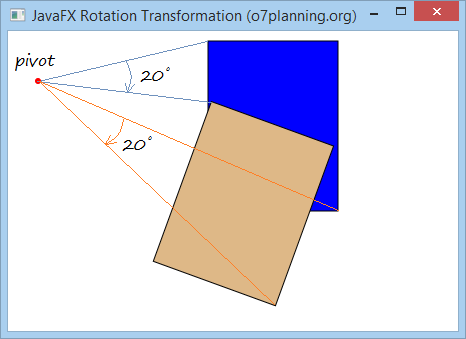RotationExample.java
``````
package org.o7planning.javafx.transformation;

import javafx.application.Application;
import javafx.scene.Group;
import javafx.scene.Scene;
import javafx.scene.paint.Color;
import javafx.scene.shape.Circle;
import javafx.scene.shape.Rectangle;
import javafx.scene.transform.Rotate;
import javafx.stage.Stage;

public class RotationExample extends Application {
@Override
public void start(Stage stage) {

final int x = 200;
final int y = 10;
final int width = 130;
final int height = 170;
int pivotX = 30;
int pivotY = 50;

// Rectangle1
Rectangle rectangle1 = new Rectangle(x, y, width, height);
rectangle1.setFill(Color.BLUE);
rectangle1.setStroke(Color.BLACK);

// Rectangle2
Rectangle rectangle2 = new Rectangle(x, y, width, height);
rectangle2.setFill(Color.BURLYWOOD);
rectangle2.setStroke(Color.BLACK);

Circle pivot = new Circle(pivotX, pivotY, 3);
pivot.setFill(Color.RED);

// Creating the rotation transformation
Rotate rotate = new Rotate();

// Setting the angle for the rotation (20 degrees)
rotate.setAngle(20);

// Setting pivot points for the rotation
rotate.setPivotX(pivotX);
rotate.setPivotY(pivotY);

// Adding the transformation to rectangle2

Group root = new Group(rectangle1, rectangle2, pivot);

Scene scene = new Scene(root, 450, 300);
stage.setTitle("JavaFX Rotation Transformation (o7planning.org)");
stage.setScene(scene);
stage.show();
}

public static void main(String args[]) {
launch(args);
}
}
``````

## 4- Scaling Transformation

Scaling Transformation is used to change the size of a geometric object. You can use it to scale the dimensions of the object.
A point will be taken as the origin of the new coordinate system. Scaling can be achieved by multiplying the original coordinates of the object with the scaling factor to get the desired result. See more illustration below.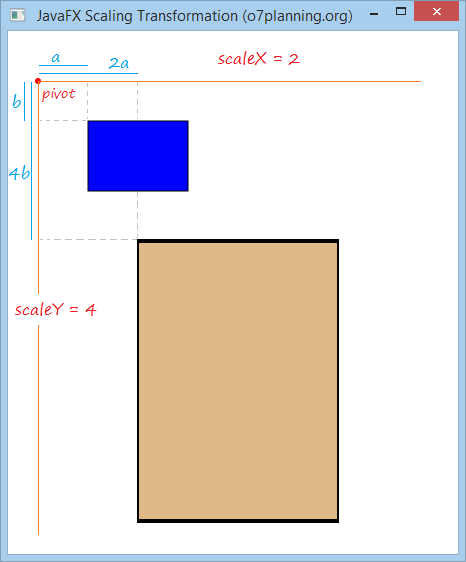For example:
There are two rectangles at the same location and in the same size. Select a point to act as origin of new coordinate system and use Scaling Transformation to scale coordinates of the object by X axis 2 times and by Y axis 4 times.
ScalingExample.java
``````
package org.o7planning.javafx.transformation;

import javafx.application.Application;
import javafx.scene.Group;
import javafx.scene.Scene;
import javafx.scene.paint.Color;
import javafx.scene.shape.Circle;
import javafx.scene.shape.Rectangle;
import javafx.scene.transform.Scale;
import javafx.stage.Stage;

public class ScalingExample extends Application {
@Override
public void start(Stage stage) {

final int x = 80;
final int y = 90;
final int width = 100;
final int height = 70;

int pivotX = 30;
int pivotY = 50;
double scaleX = 2;
double scaleY = 4;

// Rectangle1
Rectangle rectangle1 = new Rectangle(x, y, width, height);
rectangle1.setFill(Color.BLUE);
rectangle1.setStroke(Color.BLACK);

// Rectangle2
Rectangle rectangle2 = new Rectangle(x, y, width, height);
rectangle2.setFill(Color.BURLYWOOD);
rectangle2.setStroke(Color.BLACK);

Circle pivot = new Circle(pivotX, pivotY, 3);
pivot.setFill(Color.RED);

// Creating the Scale transformation
Scale scale = new Scale();

// Setting the scaliing factor.
scale.setX(scaleX);
scale.setY(scaleY);

// Setting Orgin of new coordinate system
scale.setPivotX(pivotX);
scale.setPivotY(pivotY);

// Adding the transformation to rectangle2

Group root = new Group(rectangle1, rectangle2, pivot);
Scene scene = new Scene(root, 450, 500);
stage.setTitle("JavaFX Scaling Transformation (o7planning.org)");
stage.setScene(scene);

stage.show();
}

public static void main(String args[]) {
launch(args);
}

}
``````

## 5- Shearing Transformation

Shearing - distorting an object by pushing it over in a particular direction
Parhaps you know "shear a sheep", that is an action indicating shearing a sheep. Shearing Transformation is not intended to cut part off the initial geometry. It only distorts the original image.
Shearing uses a point as pivot to transfroms an object.
If the pivot resets the origin of the coordinate system. The shearing factor of the object by X direction, and Y direction respectively is shearX, shearY, then:
• (x, y) ==> (x + y * shearY, y + x * shearX)
If the pivot point is located at the coordinate (pivotX, pivotY), and the shearing factor in the X direction, Y direction respectively is shearX, shearY, then:
• (x, y) ===> (X", Y")
• X" =  pivotX + (x-pivotX) + (y-pivotY) * shearY
• Y" =  pivotY + (y-pivotY) + (x-pivotX) * shearX
Example:
1. Assume that the pivot point is at the origin.
2. The shearing factor by X axis is shearX = 2.
3. The shearing factor by Y axis is shearY = 0.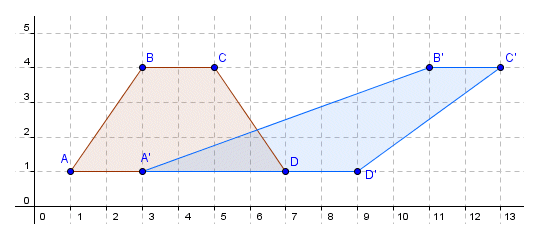We will obtain the result:
• xA" = xA + shearY * yA = 1 + 2 * 1 = 3
• yA" = yA + shearX * xA = 1 + 0 * 1 = 1
• xB" = xB + shearY * yB = 3 + 2 * 4 = 11
• yB" = yB + shearX + yB = 4 + 0 * 4 = 4
JavaFX Example:
There are two rectangles, at the same location and in the same size. Select a pivot point and use the Shearing Transformation for the second rectangle, and see the result:
ShearingExample.java
``````
package org.o7planning.javafx.transformation;

import javafx.application.Application;
import javafx.scene.Group;
import javafx.scene.Scene;
import javafx.scene.paint.Color;
import javafx.scene.shape.Circle;
import javafx.scene.shape.Rectangle;
import javafx.scene.transform.Shear;
import javafx.stage.Stage;

public class ShearingExample extends Application {

@Override
public void start(Stage stage) {
final int x = 120;
final int y = 80;
final int width = 150;
final int height = 100;

int pivotX = 40;
int pivotY = 50;
double shearX = 0.2;
double shearY = 0.5;

// (all)
// Rectangle1
Rectangle rectangle1 = new Rectangle(x, y, width, height);
rectangle1.setFill(Color.BLUE);
rectangle1.setStroke(Color.BLACK);

// (all)
// Rectangle2
Rectangle rectangle2 = new Rectangle(x, y, width, height);
rectangle2.setFill(Color.BURLYWOOD);
rectangle2.setStroke(Color.BLACK);

Circle pivot = new Circle(pivotX, pivotY, 3);
pivot.setFill(Color.RED);

// Creating the Shearing transformation
Shear shear = new Shear();

// Setting the pivot point
shear.setPivotX(pivotX);
shear.setPivotY(pivotY);

// Setting the shearing factor
shear.setX(shearX);
shear.setY(shearY);

// Adding the transformation to rectangle2

Group root = new Group(rectangle1, rectangle2, pivot);
Scene scene = new Scene(root, 450, 300);
stage.setTitle("Shearing Transformation (o7planning.org)");
stage.setScene(scene);
stage.show();
}

public static void main(String args[]) {
launch(args);
}
}
``````
Run the example: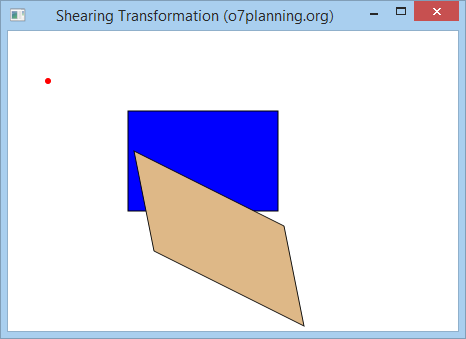Edit the above example with:
• double shearX = 0;
• double shearY = 0.5;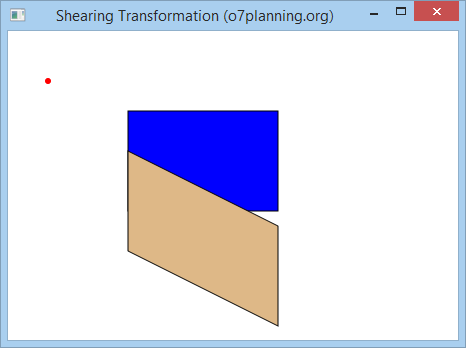Edit the above example with the values:
• double shearX = 0.2;
• double shearY = 0;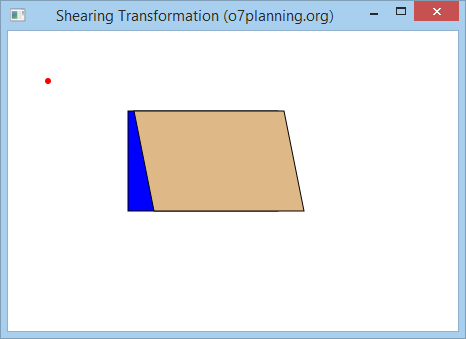## 6- Affine Transformation

Affine means a linear transformation that transforms an object from a two or three-dimensional space into another two or three-dimensional space and still keeps the straightness and parallelness of lines unchanged.
One point A with the coordinate (x, y, z) in the three-dimensional space will transfer to position A" with the coordinate (x", y", z") by a matrix multiplication: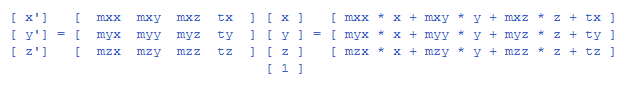The Affine class in the JavaFX has some Constructors. Below are common structructors:
``````
// Constructor to create 2D Affine.
public Affine(double mxx, double mxy, double tx,
double myx, double myy, double ty);

// Constructor to create 3D Affine.
public Affine(double mxx, double mxy, double mxz, double tx,
double myx, double myy, double myz, double ty,
double mzx, double mzy, double mzz, double tz) ;
``````
Example:
AffineExample.java
``````
package org.o7planning.javafx.transformation;

import javafx.application.Application;
import javafx.scene.Group;
import javafx.scene.Scene;
import javafx.scene.paint.Color;
import javafx.scene.shape.Circle;
import javafx.scene.shape.Rectangle;
import javafx.scene.transform.Affine;
import javafx.stage.Stage;

public class AffineExample extends Application {

@Override
public void start(Stage stage) {

final int x = 80;
final int y = 90;
final int width = 100;
final int height = 70;

double mxx = 0.2;
double mxy = 1.5;
double myx = 1.5;
double myy = 0.5;

double tx = 40;
double ty = 50;

// Rectangle1
Rectangle rectangle1 = new Rectangle(x, y, width, height);
rectangle1.setFill(Color.BLUE);
rectangle1.setStroke(Color.BLACK);

// Rectangle2
Rectangle rectangle2 = new Rectangle(x, y, width, height);
rectangle2.setFill(Color.BURLYWOOD);
rectangle2.setStroke(Color.BLACK);

Circle pivot = new Circle(tx, ty, 3);
pivot.setFill(Color.RED);

// Creating the 2D Affine transformation
Affine affine = new Affine(mxx, mxy, tx, myx, myy, ty);

// Adding the transformation to rectangle2

Group root = new Group(rectangle1, rectangle2, pivot);
Scene scene = new Scene(root, 450, 500);
stage.setTitle("JavaFX Affine Transformation (o7planning.org)");
stage.setScene(scene);

stage.show();
}

public static void main(String args[]) {
launch(args);
}

}
``````
Run the example: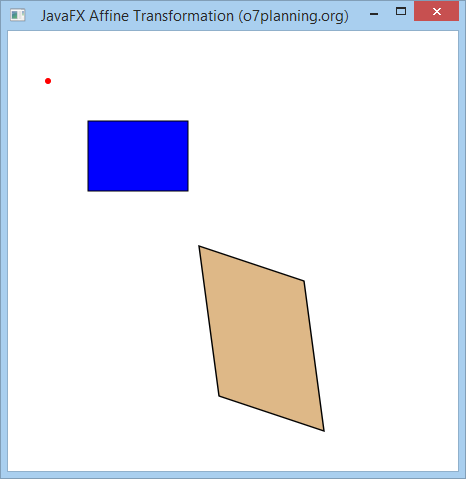## 7- Combination of transformations

You can apply many transformations to a geometric object:
Example:
In this example, you have 3 rectangles at the same location and in the same size. Create two transformations such as Rotation and Translation. Add the Rotation to the second rectangle and both above transformations to the third rectangle and see the result.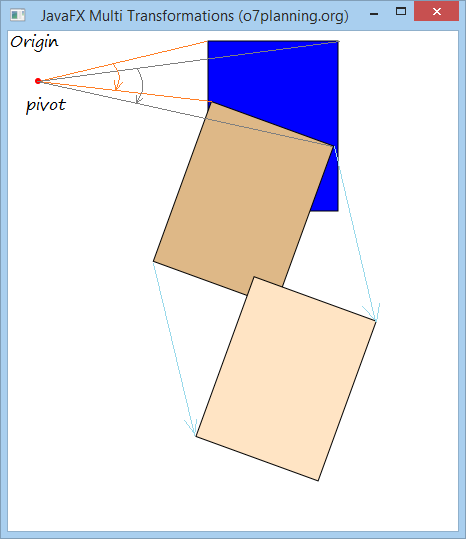MultipleTransformationsExample.java
``````
package org.o7planning.javafx.transformation;

import javafx.application.Application;
import javafx.scene.Group;
import javafx.scene.Scene;
import javafx.scene.paint.Color;
import javafx.scene.shape.Circle;
import javafx.scene.shape.Rectangle;
import javafx.scene.transform.Rotate;
import javafx.scene.transform.Translate;
import javafx.stage.Stage;

public class MultipleTransformationsExample extends Application {
@Override
public void start(Stage stage) {

final int x = 200;
final int y = 10;
final int width = 130;
final int height = 170;
int pivotX = 30;
int pivotY = 50;

// Rectangle1
Rectangle rectangle1 = new Rectangle(x, y, width, height);
rectangle1.setFill(Color.BLUE);
rectangle1.setStroke(Color.BLACK);

// Rectangle2
Rectangle rectangle2 = new Rectangle(x, y, width, height);
rectangle2.setFill(Color.BURLYWOOD);
rectangle2.setStroke(Color.BLACK);

// Rectangle3
Rectangle rectangle3 = new Rectangle(x, y, width, height);
rectangle3.setFill(Color.BISQUE);
rectangle3.setStroke(Color.BLACK);

Circle pivot = new Circle(pivotX, pivotY, 3);
pivot.setFill(Color.RED);

// Creating the rotation transformation
Rotate rotate = new Rotate();

// Setting the angle for the rotation (20 degrees)
rotate.setAngle(20);

// Setting pivot points for the rotation
rotate.setPivotX(pivotX);
rotate.setPivotY(pivotY);

// Adding the transformation to rectangle2

// Create Translation Transformation.
Translate translate = new Translate(100, 150);

// Adding 2 transformations to rectangle3

Group root = new Group(rectangle1, rectangle2,rectangle3, pivot);

Scene scene = new Scene(root, 450, 500);
stage.setTitle("JavaFX Multi Transformations (o7planning.org)");
stage.setScene(scene);
stage.show();
}

public static void main(String args[]) {
launch(args);
}
}
``````

#### View more Tutorials:

Maybe you are interested

These are online courses outside the o7planning website that we introduced, which may include free or discounted courses.

•AngularJs Practical Session with Basic To Expert Level
•JavaFX tutorial: Learn JavaFX with Examples
•C, C++, Java; A Programming MegaPrimer for Beginners
•JavaFx Tutorial For Beginners
•Sharepoint: SPFx Development Model
•JavaFX : Learn to build powerful client applications
•Mastering AngularJS UI Development
•Java for Intermediate Users
•Learning Dart: Become more productive with Dart
•Oracle SQL Developer : Essentials, Tips and Tricks
•Starting 2D Game Development in Unity with C#
•Advanced Java programming with JavaFx: Write an email client
•LEARNING PATH: SQL: Securing Data with SQL Server on Linux
•Tic-Tac-Toe Clone - The Complete SFML C++ Game Course
•Java Spring and Hibernate:create a crud application
•Learn Database Design using PostgreSQL
•Java Fx Concepts With Practical Examples
•From 0 to 1: JavaFX and Swing for Awesome Java UIs
•UI&UX Design , Animation And Material design In Javafx
•Backup and Restore Fundamentals in PostgreSQL DB - Level 2
•Build Outstanding Java Apps with JavaFX much faster
•Learn the basics of C++ fast! Learn how to Develop Software
•HTML5 for Beginners
•New to Python Automation..?Try Step by Step Python 4 Testers
•*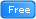* Crash Course Into JavaFX: The Best Way to make GUI Apps